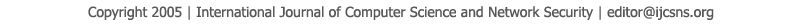To search, Click below search items.All Published Papers Search Service
 Title Author Year Keyword
 Title Neural Network Fuzzy Learning Vector Quantization (FLVQ) to Identify Probability Distributions Author Warsono, Gerry Alfa D, Dian Kurniasari, Mustofa Usman Citation Vol. 16  No. 10  pp. 16-19 Abstract A Statistical model is built based on a probability distribution. Classically, probability distribution is identified by some methods for example by using Chi-square goodness of fits, by using graph, by nonparametric goodness of fits test, and by using normal plot to test the normality. The aim of this study is going to discuss the applications of Fuzzy Learning Vector Quantization (FLVQ) model to identify some probability distributions this model is a merger between neural network and fuzzy set. The results from the application of this FLVQ through a simulation to identify the probability distributions are very good and can be implemented to a real data. Keywords neural network, fuzzy set, FLVQ, codebook vector, goodness of fits test URL http://paper.ijcsns.org/07_book/201610/20161003.pdf